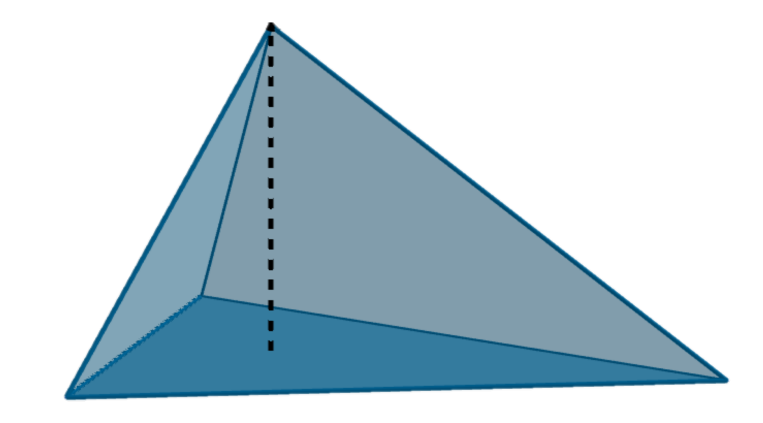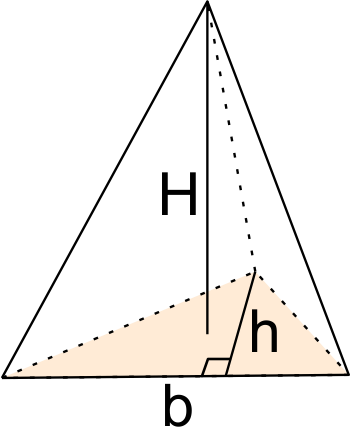# Triangular Pyramid Volume Calculator

Created by Anna Szczepanek, PhD
Reviewed by Bogna Szyk and Jack Bowater
Last updated: Mar 11, 2022

Our triangular pyramid volume calculator allows you to find the volume of any pyramid with a triangular base, regardless of whether or not you know the area of its base. If you want to determine the volume of a right triangular pyramid or a regular tetrahedron, you've come to the right place.
Do you need to learn how to find the volume of a triangular pyramid by hand? Keep reading as we give both the formula as well as examples of how to use it.

## How to use our triangular pyramid volume calculator?

A triangular pyramid is a solid object formed by connecting a triangular base to a point, called the apex. This creates four faces, each of which is a triangle. If you can rotate the pyramid, each face can play the role of the pyramid's base. The segment that is perpendicular to the base and runs through the apex is known as the height of the pyramid.

Here the dark blue triangle is the base of the pyramid and the dotted black segment is the height:To use our triangular pyramid volume calculator, follow these steps:

1. Do you know the area of the pyramid's base?
• If so, enter it into the calculator.
• If not, check what data about the base you do know: choose the appropriate option in our calculator and enter your data. The base area will be calculated for you.
1. Enter the pyramid's height.

2. The triangular pyramid volume calculator will then return your pyramid's volume :)

## Triangular pyramid volume formula

The triangular pyramid volume formula resembles the volume formula for any other pyramid or cone:

V = A * H / 3

where:

• A is the area of the pyramid's base
• H is the height from the base to the apex

In words: the volume of a triangular pyramid is one-third of the product of the base area and the pyramid's height.

## How to find the volume of a triangular pyramid by hand?

This is the part of the text where we show you how to apply the triangular pyramid volume formula. As an example, consider a triangular pyramid with height of 10 cm, and a base with sides that are 3 cm, 4 cm, and 5 cm in length (making it an Egyptian right-angled triangle):

1. Determine the area of the base: the area of the Egyptian triangle can be computed as 3 * 4 / 2 = 6
2. Find the pyramid's height: in our case, it is equal to 10
3. Apply the triangular pyramid volume formula: 6 * 10 / 3 = 20

The hardest step is usually calculating the base area - to learn more about it, you may want to check our generic triangle area calculator, or one of the calculators dedicated to each special type of triangle, be it
equilateral,
isosceles, or
right-angled (or one of the right-angled triangle's special instances,
the 45 45 90 triangle or the
30 60 90 triangle).

If you need to know how to find the volume of a triangular pyramid that is a regular tetrahedron, or a right triangular pyramid, then check out the two remaining sections where we give the appropriate formulae.

## Volume of a tetrahedron

A triangular pyramid that has equilateral triangles as its faces is called a regular tetrahedron.The volume of a tetrahedron with side of length a can be expressed as:

V = a³ * √2 / 12,

which is approximately equal to V = 0.12 * a³.

For instance, the volume of a tetrahedron of side 10 cm is equal to

V = 6³ * √2 / 12 = 18 √2,

which can be approximated as 0.12 * 6³ = 25.92.

## Volume of a right triangular pyramid

A right triangular pyramid is a triangular pyramid which has an equilateral base, and whose apex is directly above the base's centroid (its center of mass), which in this case coincides with the orthocenter (the point where all three altitudes intersect).

• The volume of a right triangular pyramid with a side of length a and height H is given by:

V = a2 * H * √3 / 12

• With help of the Pythagorean theorem, we can find the volume of a right triangular pyramid with a base of side length a, and where the edges between the base and the apex have length b. The formula reads:

V = √(b2 - a2 / 3) * a2 * √3 / 12

Anna Szczepanek, PhD
I don't know the area of the pyramid's base
Triangle type
base and heightBase (b)
in
Base height (h)
in
Pyramid base area
in²
Pyramid height (H)
in
Volume
cu in
People also viewed…

### Car vs. Bike

Everyone knows that biking is awesome, but only this Car vs. Bike Calculator turns biking hours into trees! 🌳

### Coffee kick

A long night of studying? Or maybe you're on a deadline? The coffee kick calculator will tell you when and how much caffeine you need to stay alert after not sleeping enough 😀☕ Check out the graph below!

### Isosceles right triangle hypotenuse

This isosceles right triangle hypotenuse calculator will help you calculate the sides and angles of a triangle that is both an isosceles and a right-angle triangle.

### Triangle length

Find the length of sides or magnitude of angles using our triangle length calculator.